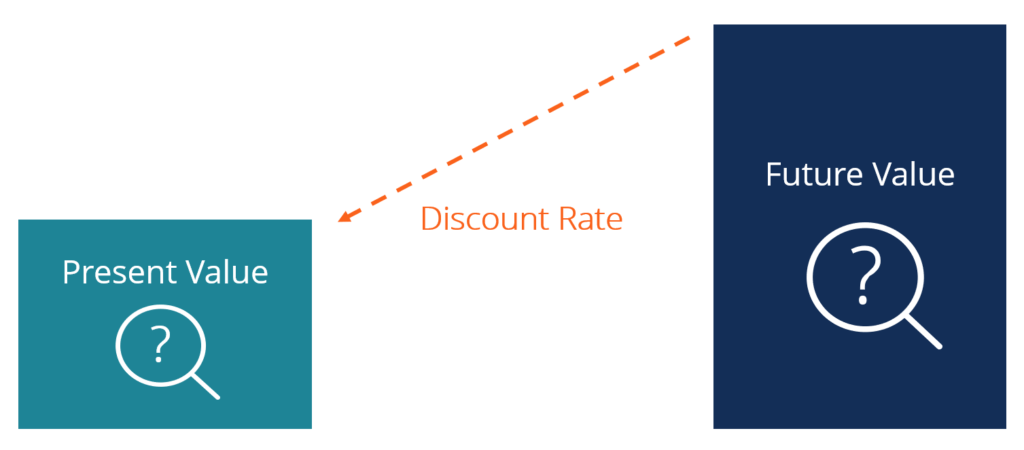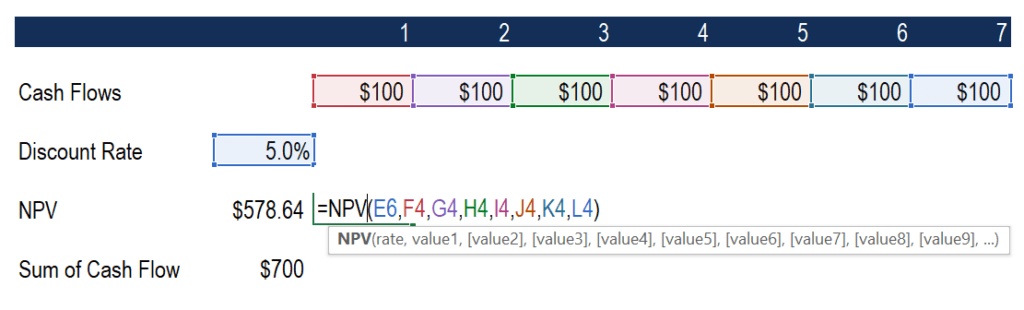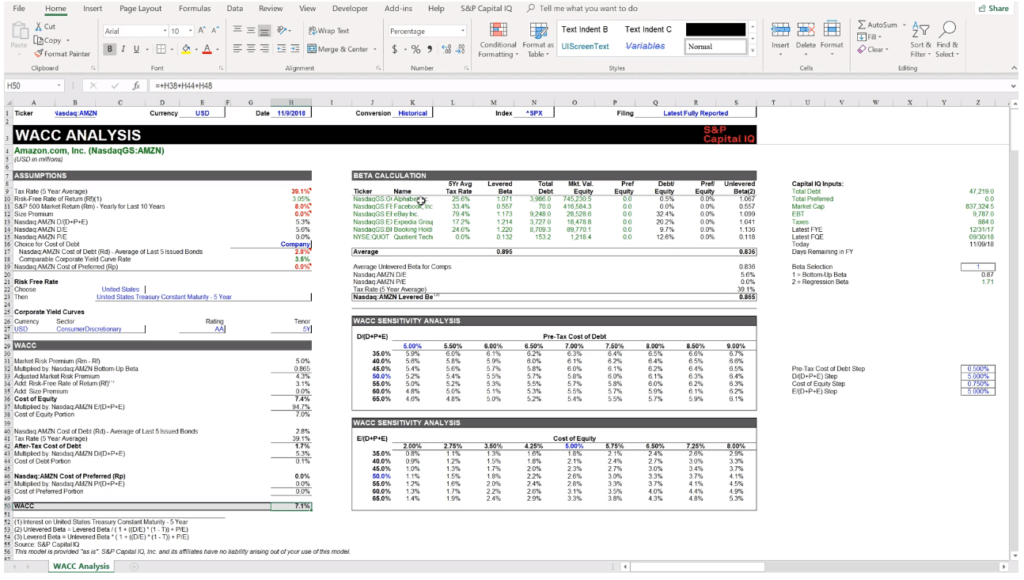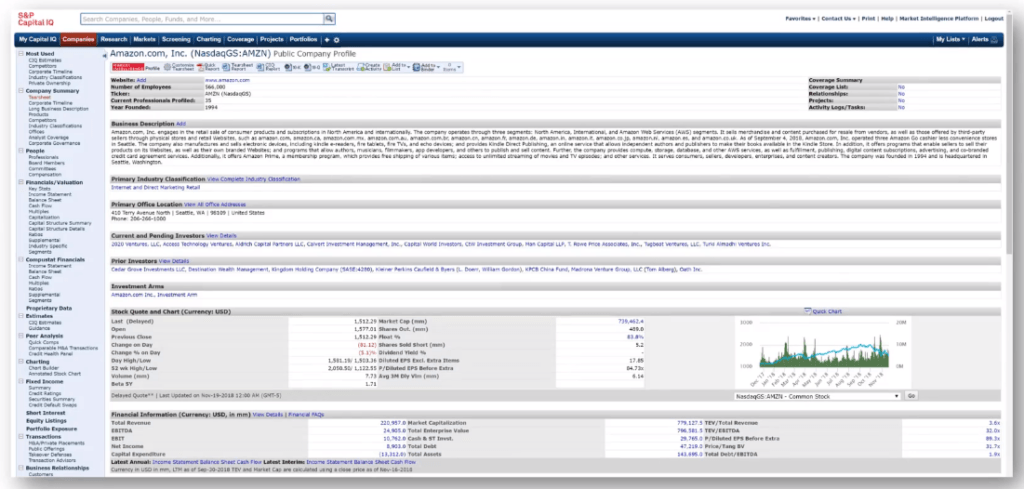# Discount Rate

A discount rate is the rate of return used to discount future cash flows back to their present value

## What is a Discount Rate?

In corporate finance, a discount rate is the rate of return used to discount future cash flows back to their present value. This rate is often a company’s Weighted Average Cost of Capital (WACC), required rate of return, or the hurdle rate investors expect to earn relative to the risk of the investment.Other types of discount rates include the central bank’s discount window rate and probability-based risk adjustments.

### Why is a Discount Rate Used?

A discount rate is used to calculate the Net Present Value (NPV) of a business as part of a Discounted Cash Flow (DCF) analysis. It is also utilized to:

• Account for the time value of money
• Account for the riskiness of an investment
• Represent the opportunity cost for a firm
• Act as a hurdle rate for investment decisions
• Make different investments more comparable

### Types of Discount Rates

In corporate finance, there are only a few types of discount rates that are used to discount future cash flows back to the present. They include:

• Weighted Average Cost of Capital (WACC) – for calculating the enterprise value of a firm
• Cost of Equity – for calculating the equity value of a firm
• Cost of Debt – for calculating the value of a bond or fixed-income security
• A pre-defined hurdle rate – for investing in internal corporate projects
• Risk-Free Rate – to account for the time value of money

### Discount Rate Example (Simple)

Below is a screenshot of a hypothetical investment that pays seven annual cash flows with each payment equal to \$100. In order to calculate the net present value of the investment, an analyst uses a 5% hurdle rate and calculates a value of \$578.64. This compares to an undiscounted total cash flow of \$700.

Essentially, an investor is saying “I am indifferent between receiving \$578.64 all at once today and receiving \$100 a year for 7 years.” This statement takes into account the investor’s perceived risk profile of the investment and an opportunity cost that they can earn on a similar investment.Below is an example from CFI’s financial modeling course on Amazon. As you can see in the screenshot, a financial analyst uses an estimate of Amazon’s WACC to discount its projected future cash flows back to the present.By using WACC to discount cash flows, the analyst is taking into account the estimated required rate of return expected by both equity and debt investors in the business.

### WACC Example

Below is a screenshot of an S&P Capital IQ template that was used in CFI’s Advanced Financial Modeling Course to estimate Amazon’s WACC.### Issues with Discount Rates

While the calculation of discount rates and their use in financial modeling may seem scientific, it is in fact not. There are so many assumptions that are only a “best guess” about what will happen in the future.

Furthermore, only one discount rate is used at a point in time to value all future cash flows, when in fact, interest rates and risk profiles are constantly changing in a dramatic way.

When using WACC as a discount rate, the calculation centers around the use of a company’s beta, which is a measure of the historical volatility of returns for an investment. The historical volatility of returns is not necessarily a good measure of how risky something will be in the future.

CFI offers the Financial Modeling & Valuation Analyst (FMVA)™ certification program for those looking to take their careers to the next level. To keep learning and advancing your career, the following resources will be helpful:

• Coupon Rate
• Internal Rate of Return (IRR)
• Unlevered Beta
• Valuation Methods

### Financial Analyst Training

Get world-class financial training with CFI’s online certified financial analyst training program!

Gain the confidence you need to move up the ladder in a high powered corporate finance career path.

Learn financial modeling and valuation in Excel the easy way, with step-by-step training.